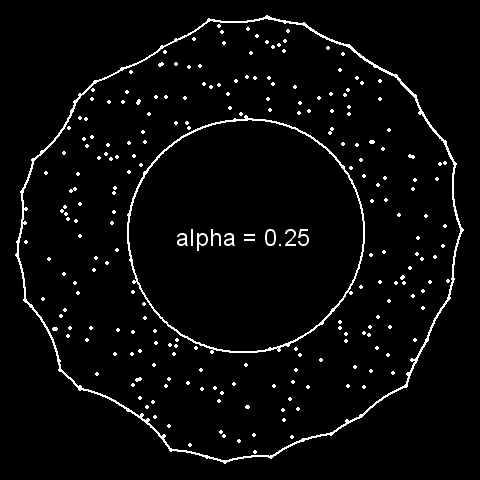# alphahull: an R Package for Alpha-Convex Hull

### Yihui Xie 2010-04-17

A new paper on the α-convex hull appeared in the Journal of Statistical Software today. The α-convex hull is an interesting problem which caught my attention long time ago but I didn’t know a solution then. R has a function `chull()` which can generate (indices of) the convex hull for a series of points. Now we can use the R package alphahull to compute the α-convex hull. For those who are not familiar with the α-convex hull, the animation below might be a good illustration for the difference between a convex hull and an α-convex hull. Note how the parameter α affects the shape of the hull:The above animation can be reproduced with the code below (uncomment the lines to create a GIF animation with the animation package):

``````set.seed(123)
theta = runif(n <- 300, 0, 2 * pi)
r = sqrt(runif(n, 0.25^2, 0.5^2))
x = cbind(0.5 + r * cos(theta), 0.5 + r * sin(theta))

## library(animation)
## saveMovie({
library(alphahull)
par(
mar = rep(0, 4), xaxt = "n", yaxt = "n", bg = "black",
col = "white"
)
for (alpha in seq(0.25, 0, -0.01)) {
plot(ahull(x, alpha = alpha), pch = 20, col = "white",
panel.last = text(
0.5, 0.5, sprintf("alpha = %.2f", alpha), cex = 2
)
)
}
## }, moviename = "alpha-convex-hull")
``````

Again, you may interactively play with the convex hull using the gWidgets package:

``````## install.packages('gWidgetsRGtk2') first if not installed
library(gWidgetsRGtk2)
options(guiToolkit = "RGtk2")

g = glayout(container = gwindow("alpha-convex hull demo"))
g[1, 1:2, expand = TRUE] = ggraphics(container = g)
g[2, 1] = "alpha"
g[2, 2, expand = TRUE] = gslider(
from = 0, to = .3, value = .2, by = .01,
container = g, handler = function(h, ...) {
par(mar = rep(0, 4), xaxt = "n", yaxt = "n")
plot(ahull(x, alpha = svalue(h\$obj)), pch = 20, ann = FALSE)
}
)
``````